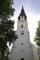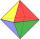Hexagonal pyramid

Calculate the volume and the surface of a regular hexagonal pyramid with a base edge length 3 cm and a height 5 cm.

Result

V =  38.971 cm3
S =  74.095 cm2

Solution:Leave us a comment of example and its solution (i.e. if it is still somewhat unclear...):Be the first to comment!To solve this example are needed these knowledge from mathematics:

Tip: Our volume units converter will help you with converion of volume units. Pythagorean theorem is the base for the right triangle calculator.

Next similar examples:

1. Hexagonal pyramidBase of the pyramid is a regular hexagon, which can be circumscribed in a circle with a radius of 1 meter. Calculate the volume of a pyramid 2.5 meters high.
2. Pyramid 4sidesCalculate the volume and the surface of a regular quadrangular pyramid when the edge of the base is 4 cm long and the height of the pyramid is 7 cm.
3. Triangular pyramidCalculate the volume and surface area of a regular triangular pyramid whose height is equal to the length of the base edges 10 cm.The regular quadrangular pyramid has a base length of 6 cm and a side edge length of 9 centimeters. Calculate its volume and surface area.
5. Wall heightCalculate the surface and volume of a regular quadrangular pyramid if side a = 6 cm and wall height v = 0.8dm.
6. Triangular pyramidDetermine the volume and surface area of a regular triangular pyramid having a base edge a=20 cm and a lateral edge b = 35 cmFind the volume and surface of a regular quadrilateral pyramid if the bottom edge is 45 cm long and the pyramid height is 7 cm.
8. Church roofThe roof of the church tower has the shape of a regular tetrahedral pyramid with base edge length 5.4 meters and a height 5 m. It was found that needs to be corrected 27% covering of the roof area. What amount of material will be required?
9. 4s pyramidRegular tetrahedral pyramid has a base edge a=17 and collaterally edge length b=32. What is its height?
10. OctahedronAll walls of regular octahedron are identical equilateral triangles. ABCDEF octahedron edges have a length d = 6 cm. Calculate the surface area and volume of this octahedron.
11. Cone area and sideCalculate the surface area and volume of a rotating cone with a height of 1.25 dm and 17,8dm side.
12. Triangular prismCalculate the surface area and volume of a triangular prism, base right triangle if a = 3 cm, b = 4 cm, c = 5 cm and height of prism h=12 cm.
13. Cube volumeThe cube has a surface of 384 cm2. Calculate its volume.
14. TetrahedronCalculate height and volume of a regular tetrahedron whose edge has a length 18 cm.
15. Theorem proveWe want to prove the sentence: If the natural number n is divisible by six, then n is divisible by three. From what assumption we started?
16. DistanceWha is the distance between the origin and the point (18; 22)?
17. Truncated cone 5The height of a cone 7 cm and the length of side is 10 cm and the lower radius is 3cm. What could the possible answer for the upper radius of truncated cone?